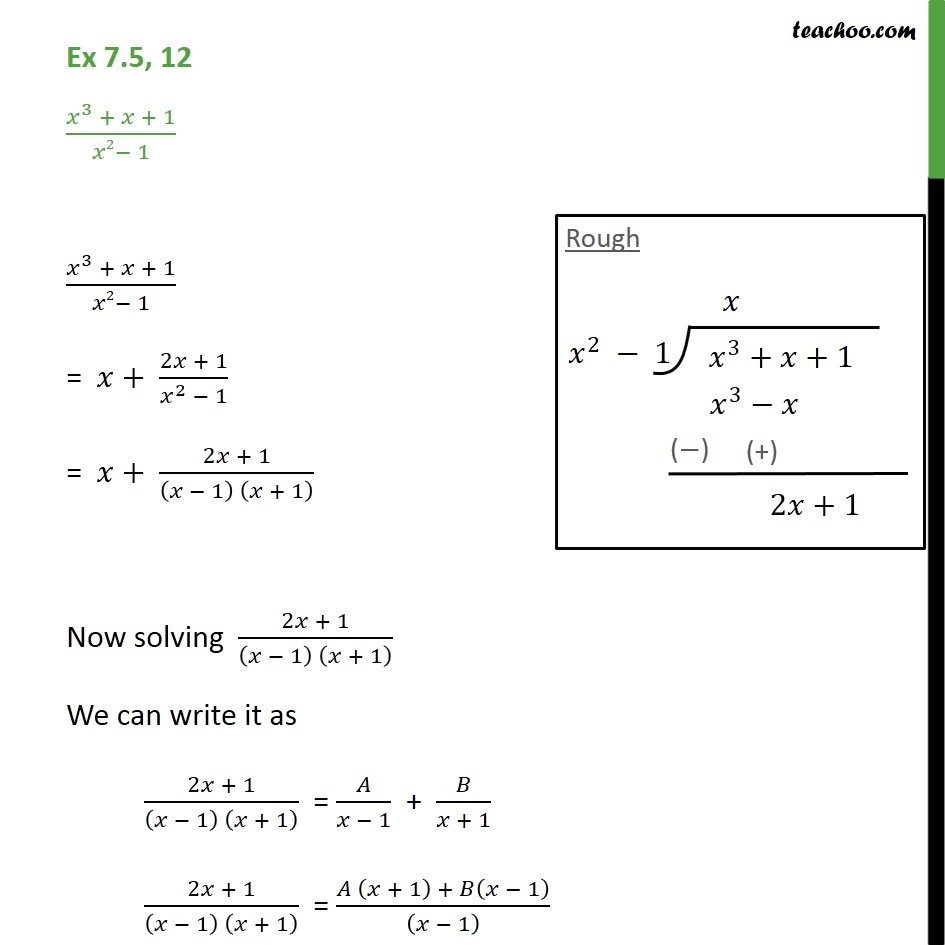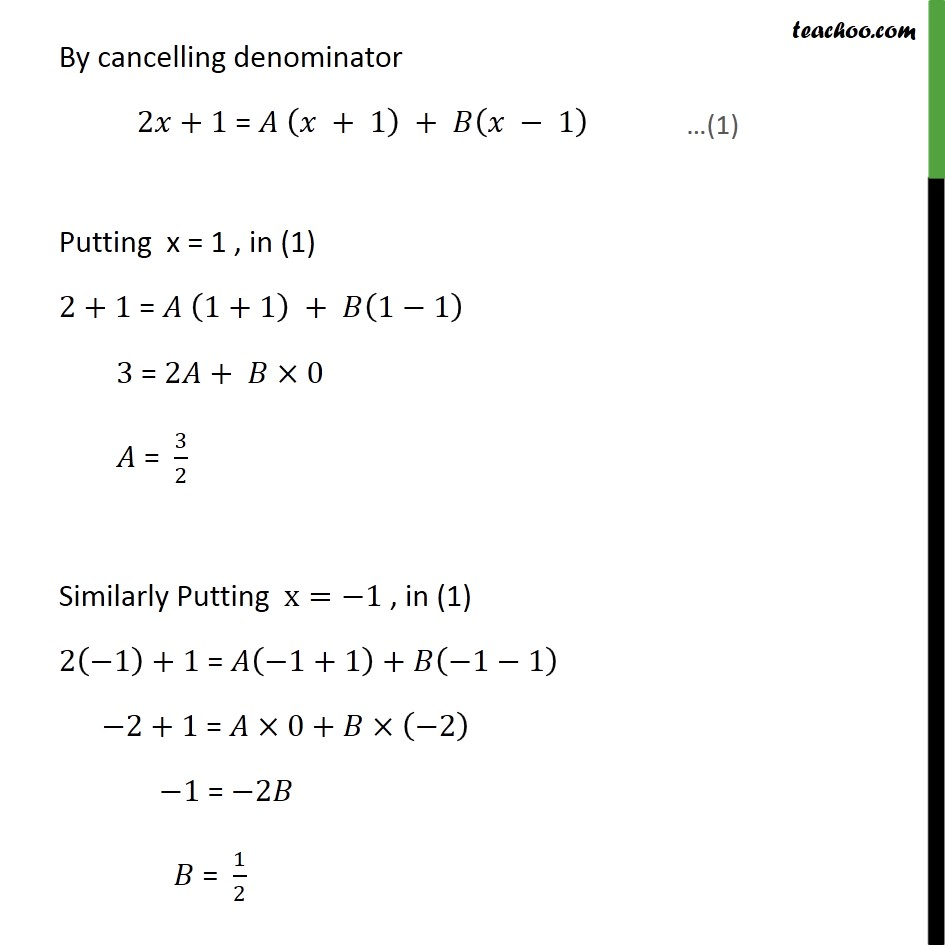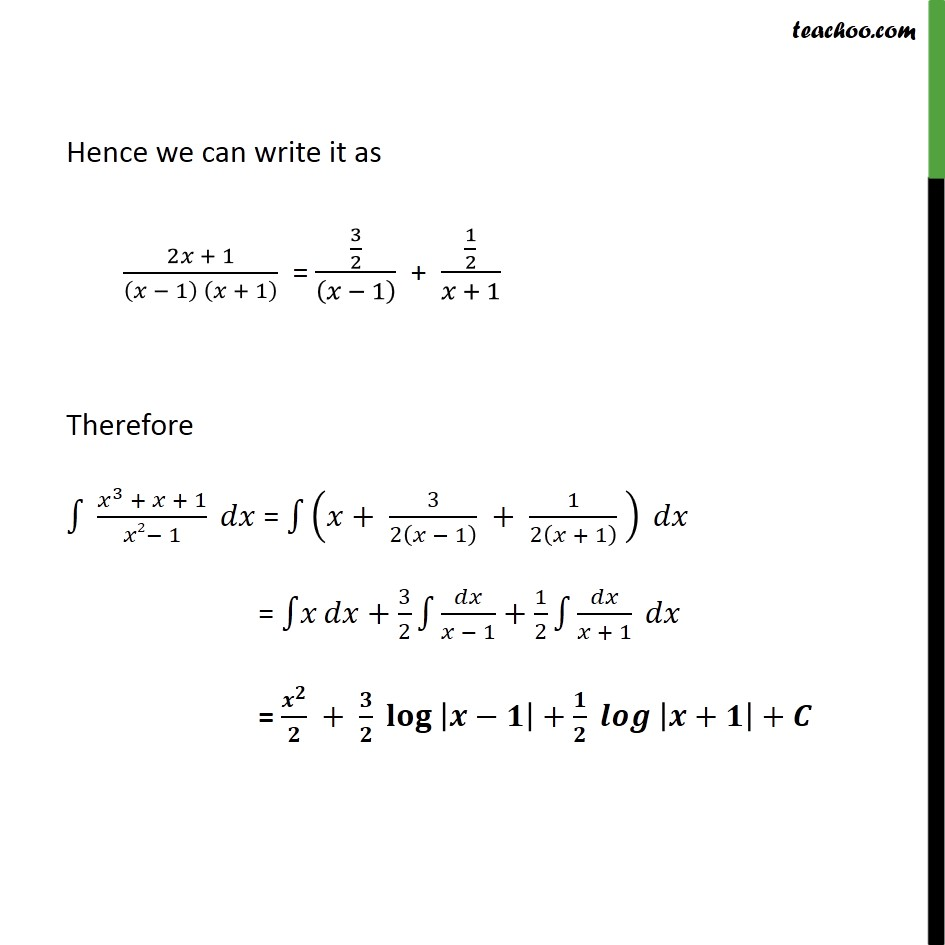Ex 7.5

Chapter 7 Class 12 Integrals
Serial order wiseLearn in your speed, with individual attention - Teachoo Maths 1-on-1 Class

### Transcript

Ex 7.5, 12 3 + + 1 2 1 3 + + 1 2 1 = + 2 + 1 2 1 = + 2 + 1 1 + 1 Now solving 2 + 1 1 + 1 We can write it as 2 + 1 1 + 1 = 1 + + 1 2 + 1 1 + 1 = + 1 + 1 1 By cancelling denominator 2 +1 = + 1 + 1 Putting x = 1 , in (1) 2+1 = 1+1 + 1 1 3 = 2 + 0 = 3 2 Similarly Putting x= 1 , in (1) 2 1 +1 = 1+1 + 1 1 2+1 = 0+ 2 1 = 2 = 1 2 Hence we can write it as 2 + 1 1 + 1 = 3 2 1 + 1 2 + 1 Therefore 3 + + 1 2 1 = + 3 2 1 + 1 2 + 1 = + 3 2 1 + 1 2 + 1 = + + + +